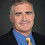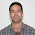## Thursday, December 13, 2018

### Inverting Onto Functions

Here's an open question that goes back to a 2003 paper  that I wrote with Steve Fenner, John Rogers and Ashish Naik. The conference paper goes back to 1996.

In that paper we discuss two hypothesis we badly called Q and Q' and it still remains open whether the two hypotheses are equivalent.

Q has a number of equivalent definitions, including

• For all NP machines M that accepting all strings, there is polynomial-time computable function f such that f(x) is an accepting path of M(x) for all x.
• For every onto honest polynomial-time computable function g there is a polynomial-time computable function f such that f finds an inverse of g, more precisely g(f(g(x))) = g(x) for all x.
• TFNP is computable in FP.
For lots more equivalences see the paper

Q' is the bit version of Q. For example

• For all NP machines M that accepting all strings, there is polynomial-time computable function f such that f(x) outputs the first bit of an accepting path of M(x) for all x.
• For every onto honest polynomial-time computable function g there is a polynomial-time computable function f such that f finds the first bit of an inverse of g, more precisely for all x there is a y such that g(y) = x and f(x) is the first bit of y.
Now Q implies Q', if you can find an accepting path of M(x) you can just read off the first bit. Does Q' imply Q?

If P = NP you can find solutions using self-reductions. For Q' self-reduction gets stuck because as you start filling in bits you may lose the "onto" promise.

On the other hand we don't even know any relativized worlds where Q' is true and Q is false. So either prove that Q' implies Q or show a relativized world where Q' is true and Q is false.

How often can I dole out 22-year old open problems that don't require deep complexity to understand. Can't promise what techniques you'll need to solve it.

1.I see that Q is interesting, I am not sure why Q' is of interest? Is this related to a known result that states computing the first/last bit of inverse of Discrete Log is as hard as inverting Discrete Log?

Thanks

1.You don't have to focus on the first bit. You get the same Q' if you use any other bit, or any polynomial-time time computable function of the witness. Q' implies that P = NP intersect co-NP so you already get factoring and discrete log as a consequence.

2.I found it strange that any polynomial time computable function of the witness works to characterize the Q', because we can use the identity function which gives the witness and this implies that Q' is equivalent to Q.

3.Good point. I meant any polynomial-time computable function with a single bit output.

2.Minor point: in the second bullet point of the description of Q', there is "more precisely for all x there is a y such that g(y) = g(x)", but should be "[...]g(y) = x", correct?

1.You are right. Now fixed. Thanks.

3.P versus NP is considered as one of the most important open problems in computer science. This consists in knowing the answer of the following question: Is P equal to NP? A precise statement of the P versus NP problem was introduced independently by Stephen Cook and Leonid Levin. Since that date, all efforts to find a proof for this problem have failed. Given a positive integer x and a collection S of positive integers, MAXIMUM is the problem of deciding whether x is the maximum of S. We prove this problem is complete for P. Another problem is SUCCINCT-MAXIMUM. SUCCINCT-MAXIMUM contains the instances of MAXIMUM that can be represented by an exponentially more succinct way. We show this succinct version of MAXIMUM must not be in P. Under the assumption of P = NP, we prove the membership of SUCCINCT-MAXIMUM in P is also hold. In this way, we demonstrate the complexity class P is not equal to NP by the reduction ad absurdum rule. Another major complexity classes are LOGSPACE and NLOGSPACE. Whether LOGSPACE = NLOGSPACE is another fundamental question that it is as important as it is unresolved. We show the problem MAXIMUM can be solved in logarithmic space. Consequently, we demonstrate the complexity class LOGSPACE is equal to P and thus, LOGSPACE is equal to NLOGSPACE.

4.You can participate in the debate

5.